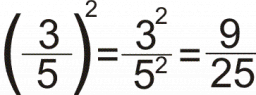# Evaluate statement

Which is a correct statement about the description “two less than the quotient of a number cubed and sixteen, increased by eight” when n = 4?

Choices:
The value when n = 4 is 6.
The value when n = 4 is 7.
The value when n = 4 is 9.
The value when n = 4 is 10.

v =  10

### Step-by-step explanation:Did you find an error or inaccuracy? Feel free to write us. Thank you!

Tips for related online calculators
Need help calculating sum, simplifying, or multiplying fractions? Try our fraction calculator.